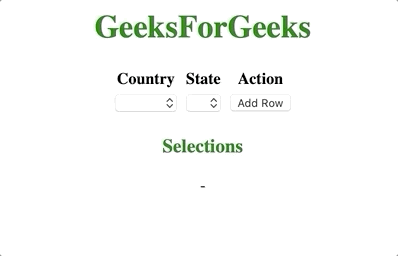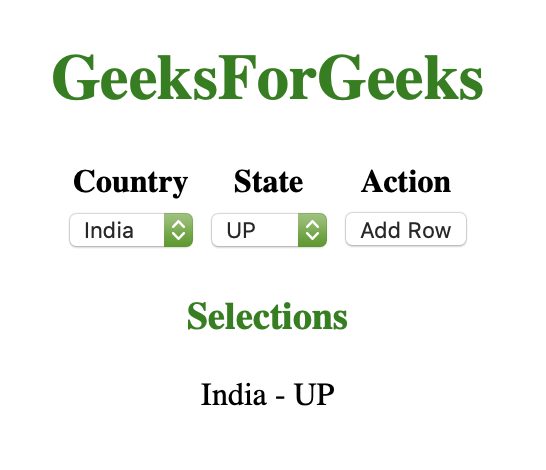# How to Add Dynamic Options for Multiple Selects Inside ng-repeat Directive ?

Given an HTML document containing some options element and the task is to add an array of javascript objects dynamically with multiple selects using ng-repeat in angularJS.

Approach: The task is done using ng-repeat which loops through an array. Let’s call this array “models”. Each select menu present in the DOM is modeled to its specific index in the array. For example, the 2nd select menu would be modeled to the 2nd object in the model’s objects array. To add more select menus to the DOM, we only need to push an empty object to the model’s array, the ng-repeat directive takes care of the rest of reproduction.

Example 1: In this example, we will add multiple selects and display the selected data.

 ` ` `<``html` `ng-app``=``"gfg"``> ` ` `  `<``head``> ` `    ``<``meta` `charset``=``"utf-8"` `/> ` `    ``<``script` `data-require``=``"angular.js@1.5.x"` `            ``src``= ` `"https://cdnjs.cloudflare.com/ajax/libs/angular.js/1.5.11/angular.min.js"` `            ``data-semver``=``"1.5.11"``> ` `    `` ` `     `  `    ``<``script``> ` `        ``var app = angular.module('gfg', []); ` ` `  `        ``app.controller('MainCtrl', function(\$scope) { ` `            ``\$scope.models = [{}]; ` `            ``\$scope.countries = ['India', 'Japan', 'US']; ` `            ``\$scope.states = { ` `                ``India: ['UP', 'MP', 'Bihar'], ` `                ``Japan: ['Tokyo', 'Yokohama'], ` `                ``US: ['California', 'Texas'], ` `            ``} ` `            ``\$scope.addRow = function() { ` `                ``\$scope.models.push({}); ` `            ``} ` `            ``\$scope.getState = function(country) { ` `                ``return \$scope.states[country]; ` `            ``} ` `        ``}); ` `    `` ` ` ` ` `  `<``body` `ng-controller``=``"MainCtrl"``> ` `    ``<``center``> ` `        ``<``h1` `style``=``"color: green;"``> ` `            ``GeeksForGeeks ` `        `` ` ` `  `        ``<``table``> ` `            ``<``tr``> ` `                ``<``th``>Country ` `                ``<``th``>State ` `                ``<``th``>Action ` `            `` ` `             `  `            ``<``tr` `ng-repeat``=``"model in models"``> ` `                ``<``td``> ` `                    ``<``select` `ng-options``= ` `                    ``"country as country for country in countries"` `                            ``ng-model``=``"model.country"` `                            ``ng-change``=``'getState(model.country)'``> ` `                    `` ` `                `` ` `                ``<``td``> ` `                    ``<``select` `ng-options``= ` `            ``"state as state for state in getState(model.country)"` `                            ``ng-model``=``"model.state"``> ` `                    `` ` `                `` ` `                ``<``td``> ` `                    ``<``button` `ng-click``=``"addRow()"``>Add Row ` `                `` ` `            `` ` `        `` ` `        ``<``h3` `style``=``"color:green"``>Selections ` `        ``<``p` `ng-repeat``=``"model in models"``> ` `            ``{{model.country}} - {{model.state}} ` `        `` ` `    `` ` ` ` ` `  ` `

Output: All the data is successfully added to the objects array.Example 2: In this example, we prepopulate the models array.

 ` ` `<``html` `ng-app``=``"gfg"``> ` ` `  `<``head``> ` `    ``<``meta` `charset``=``"utf-8"` `/> ` `    ``<``script` `data-require``=``"angular.js@1.5.x"` `            ``src``= ` `"https://cdnjs.cloudflare.com/ajax/libs/angular.js/1.5.11/angular.min.js"` `            ``data-semver``=``"1.5.11"``> ` `    `` ` `     `  `    ``<``script``> ` `        ``var app = angular.module('gfg', []); ` `         `  `        ``// Prepopulate the models array here ` `        ``app.controller('MainCtrl', function(\$scope) { ` `            ``\$scope.models = [{ ` `                ``country: 'India', ` `                ``state: 'UP' ` `            ``}]; ` `            ``\$scope.countries = ['India', 'Japan', 'US']; ` `            ``\$scope.states = { ` `                ``India: ['UP', 'MP', 'Bihar'], ` `                ``Japan: ['Tokyo', 'Yokohama'], ` `                ``US: ['California', 'Texas'], ` `            ``} ` `            ``\$scope.addRow = function() { ` `                ``\$scope.models.push({}); ` `            ``} ` `            ``\$scope.getState = function(country) { ` `                ``return \$scope.states[country]; ` `            ``} ` `        ``}); ` `    `` ` ` ` ` `  `<``body` `ng-controller``=``"MainCtrl"``> ` `    ``<``center``> ` `        ``<``h1` `style``=``"color: green;"``> ` `            ``GeeksForGeeks ` `        `` ` ` `  `        ``<``table``> ` `            ``<``tr``> ` `                ``<``th``>Country ` `                ``<``th``>State ` `                ``<``th``>Action ` `            `` ` `             `  `            ``<``tr` `ng-repeat``=``"model in models"``> ` `                ``<``td``> ` `                    ``<``select` `ng-options``= ` `            ``"country as country for country in countries"` `                        ``ng-model``=``"model.country"` `                        ``ng-change``=``'getState(model.country)'``> ` `                    `` ` `                `` ` `                ``<``td``> ` `                    ``<``select` `ng-options``= ` `         ``"state as state for state in getState(model.country)"` `                            ``ng-model``=``"model.state"``> ` `                    `` ` `                `` ` `                ``<``td``> ` `                    ``<``button` `ng-click``=``"addRow()"``>Add Row ` `                `` ` `            `` ` `        `` ` `        ``<``h3` `style``=``"color:green"``>Selections ` `        ``<``p` `ng-repeat``=``"model in models"``> ` `            ``{{model.country}} - {{model.state}} ` `        `` ` `    `` ` ` ` ` `  ` `

Output: We see that the page now always contain country “India” and state “UP” as its prepopulated on page load.Whether you're preparing for your first job interview or aiming to upskill in this ever-evolving tech landscape, GeeksforGeeks Courses are your key to success. We provide top-quality content at affordable prices, all geared towards accelerating your growth in a time-bound manner. Join the millions we've already empowered, and we're here to do the same for you. Don't miss out - check it out now!